CLIMATE TECH:

SHALL WE SAVE BY DOING SPORTS?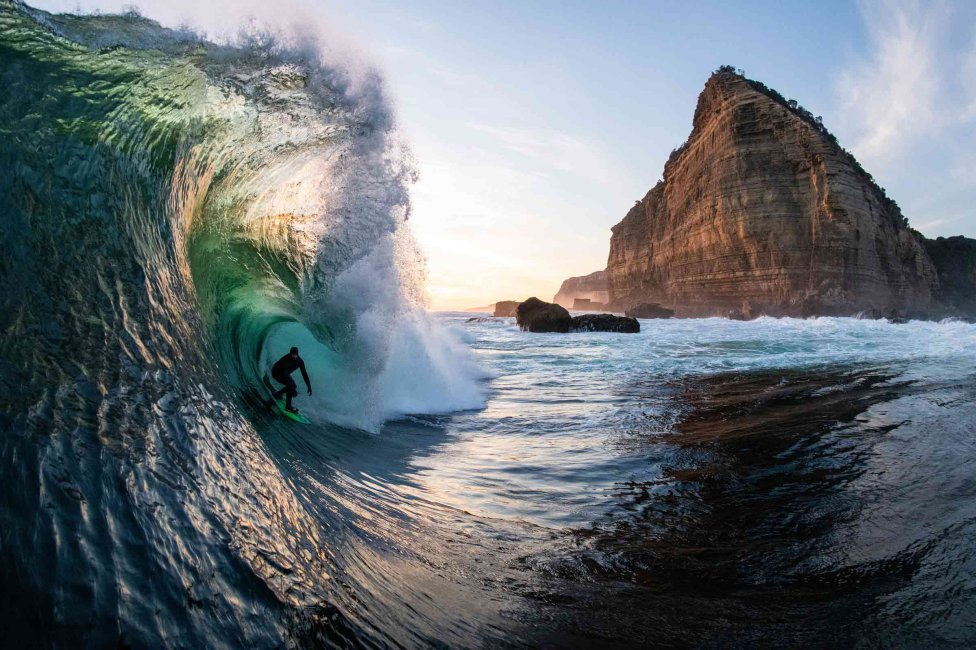“I recently had a question from some online students at the University of Hawaii, about their concern about the days when the waves didn’t satisfy them in their favorite sport.

That’s why I set out to do calculations with them to give them a viable, scalable, and low-cost solution.”

How much would it cost to drive your own wave?

When you are a surfer at heart who enjoys nature, you know you have a problem: “you can only surf where there are waves”.

Even if you are lucky enough to live near an ocean like where I was born, the waves to surf are not consistent on a daily basis.

It can be perfect to take a day, the rest is perfect, and the next day the water is flat as glass.

Well, there is a simple solution to this problem:

EXIST!

That’s exactly what happens at places like surf ranch, which has created a massive wave that travels 2.300 feet.

Making an artificial wave machine starts with a body of water.

In this case, it’s basically a rectangular pond.

On one of the long sides is a type of rail car with a hydrofoil, essentially a wing that runs across the water.

As the hydrofoil moves, it creates waves to ride on.

Also, by adjusting the hydrofoil, the waves can be customized (to a degree) to produce waves between 6 and 8 feet high with speeds between 10 and 20 miles per hour.

COMMON PROBLEMS AND SOLUTIONS

But let’s say you don’t live near any surf park and you decide to build your own.

Let’s not worry about the technical details.

We’ll assume you already have a small lake and can build a track next to it that will push your giant hydrofoil powered by an electric motor. Instead, let’s get to the real question:

How much is a single wave going to cost you?

This can teach you how to memorize anything

When you pay your electric bill each month, you are paying for energy in the form of electric current.

Electricity prices vary by location, but we can estimate the amount of power needed to create a fake wave, and then use average prices to figure out how much it would cost.

Imagine a very simple wave.

If you look at it from the side, it could take a form like this: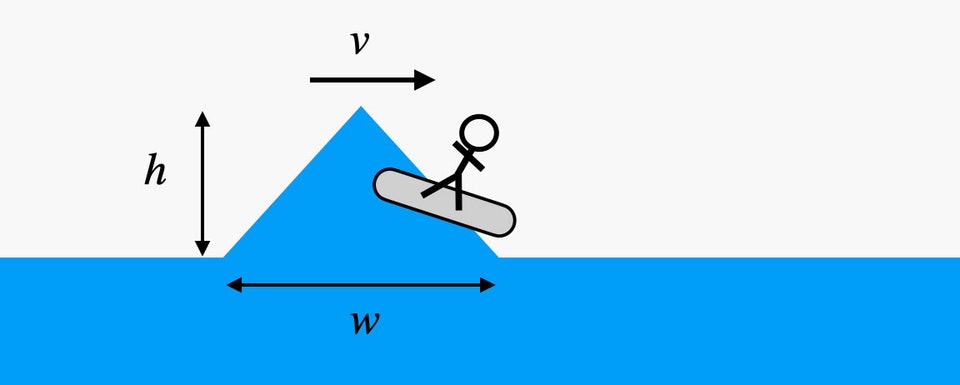The actual dimensions are not important, but we are going to need some values ​​for our calculation. The simple triangular wave with height h and width W.

You can’t see it in the picture, but the wave also has a length, which is probably the same width as the lake.

Let’s call that L.

Finally, there is the speed of the wave, which will be represented by V. (In the image, the wave travels to the right.)

This simple wave will have two types of energy once it is in motion: kinetic energy and gravitational potential energy.

Kinetic energy is associated with the motion of an object (the wave, in this case), and it depends on both the mass of the object and its speed.

We can calculate the kinetic energy with the following equation:

(K is equal to half of M times V squared.)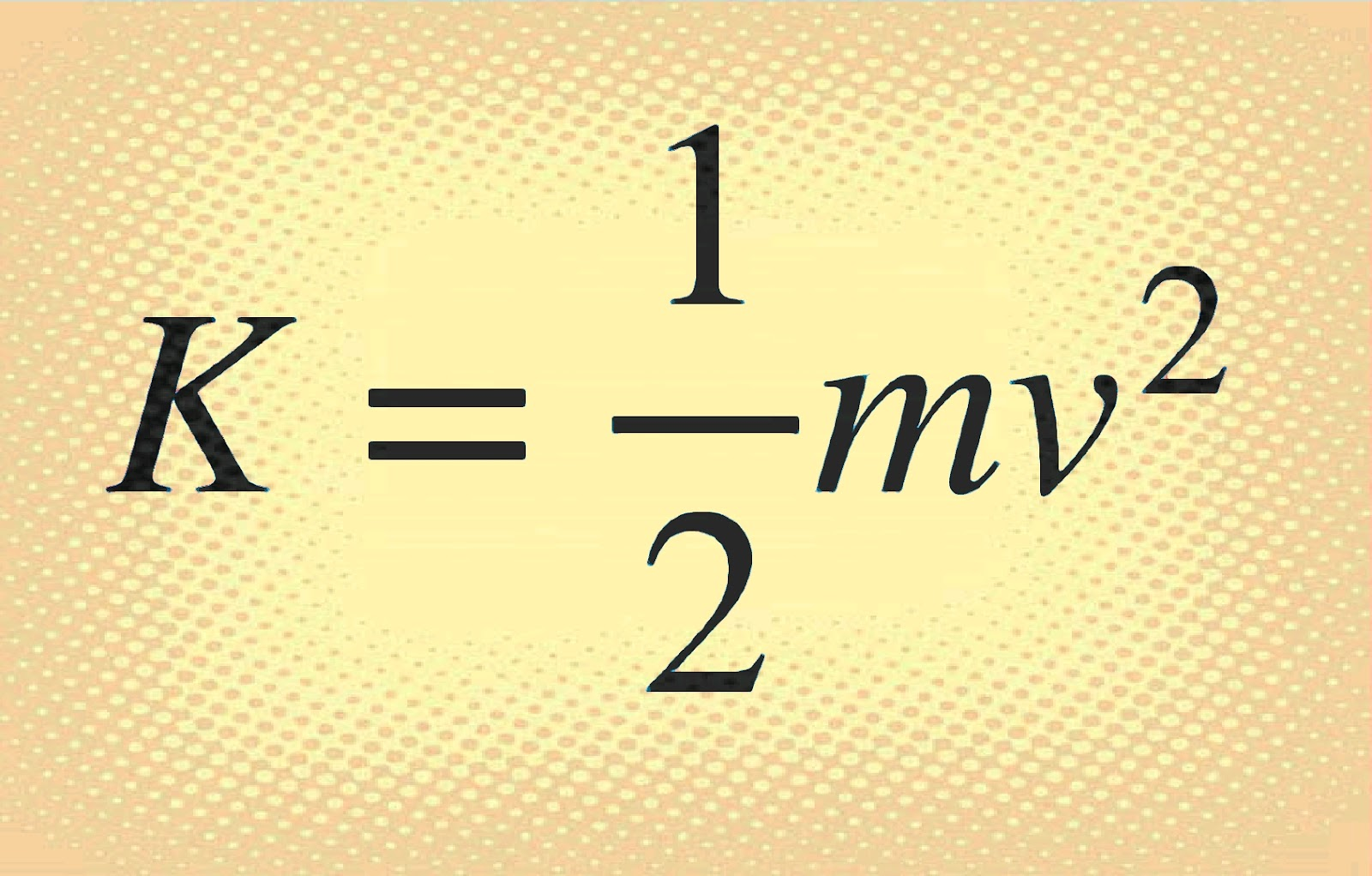Yeah, we don’t know the mass of the wave yet, wait.

The best is coming!.

The other type of energy that the wave has is gravitational potential energy.

This is associated with the gravitational interaction between water and Earth, since as an object moves away from the Earth’s surface, it has an increase in gravitational potential energy.

Since this wave is protruding above the surface of the water, it will have some potential.

We almost always use U to represent potential energy, and we can calculate it like this:

(U equals M times G times H).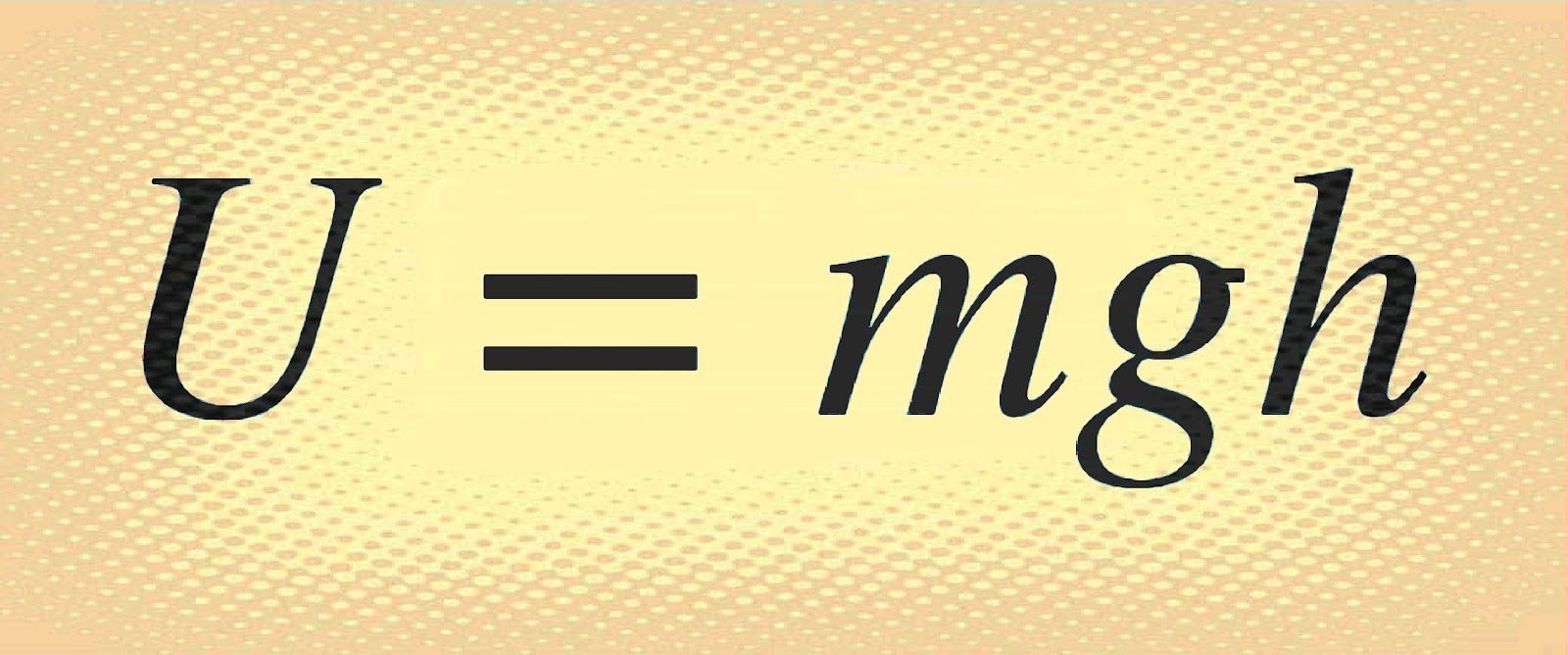That is the gravitational field.

It is a measure of the strength of the gravitational interaction.

On the Earth’s surface it has a value of 9.8 newtons per kilogram.

If you want to build your surf park on another planet, there will be a different value for the gravitational field.

For example, on Mars, G = 3.75 N/kg due to the weaker gravitational interaction.

Be careful with the height (h) in this equation, since different parts of the wave are at different heights above the surface.

Since it is a triangular wave, most of the water is near the surface and only a small part is on top.

Instead of using the height of the triangle wave, we can use the height of the center of mass of the wave.

Fortunately, since this is a triangle, we know that the center of mass would be 1/3 of the height of the wave.

Nice!.

THE IMPORTANT DATA

Both kinetic and gravitational potential energy depend on the mass of the wave.

Assuming that the wave is made of water (there are other options to consider), then we know that the density is 1.000 kilograms per cubic meter.

Now we just need to find the wave volume (V) to determine the mass.

Since this simple wave is just a triangular prism, I can find the volume with no problem.

Together with the density ( ρ ), I can get the mass, like this:

(Mass equals P times V P equals mass over V equals half W times H times L.)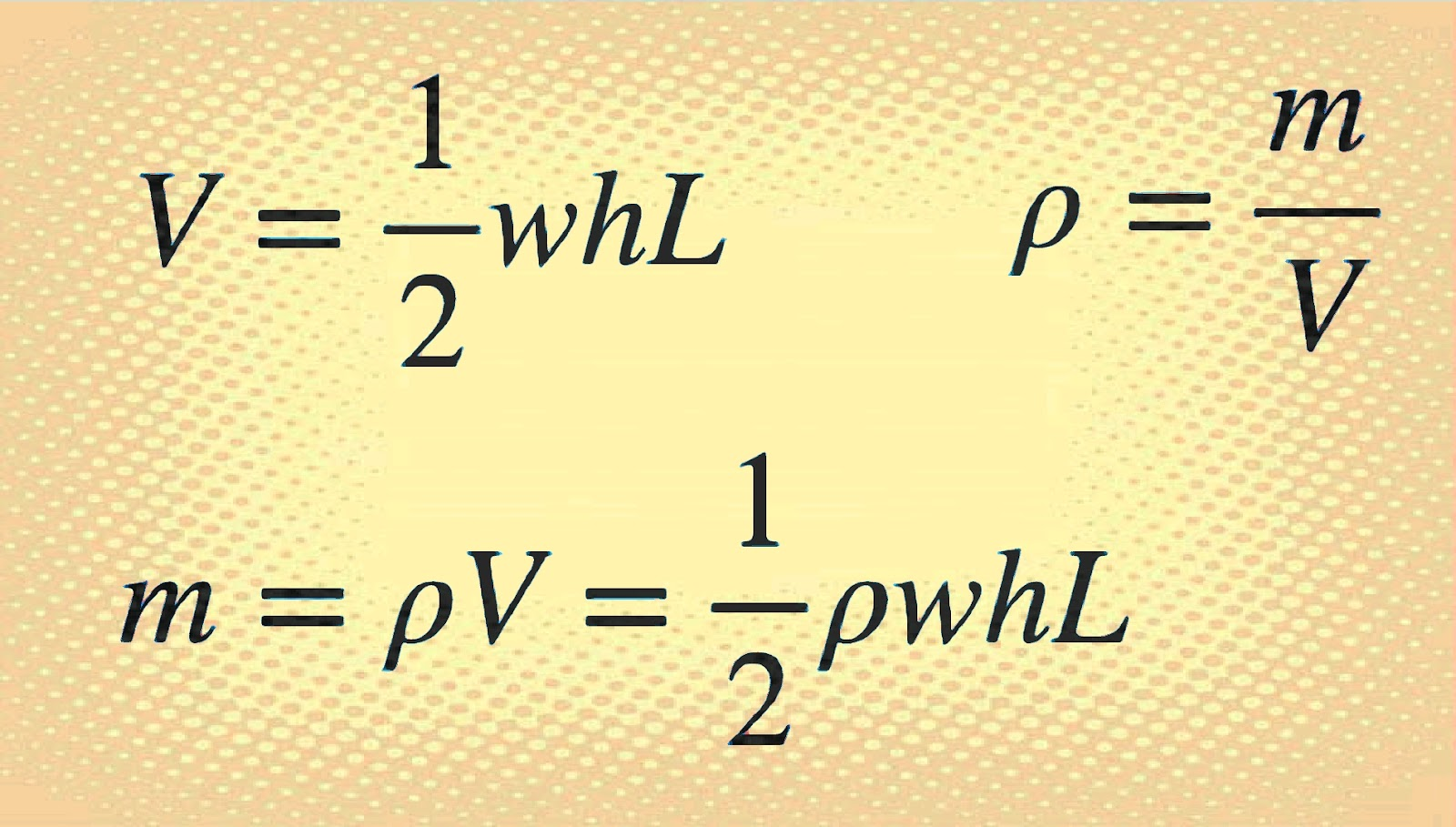Putting all this together, we get the following expression for the total energy of a wave:

WAVE EQUATIONS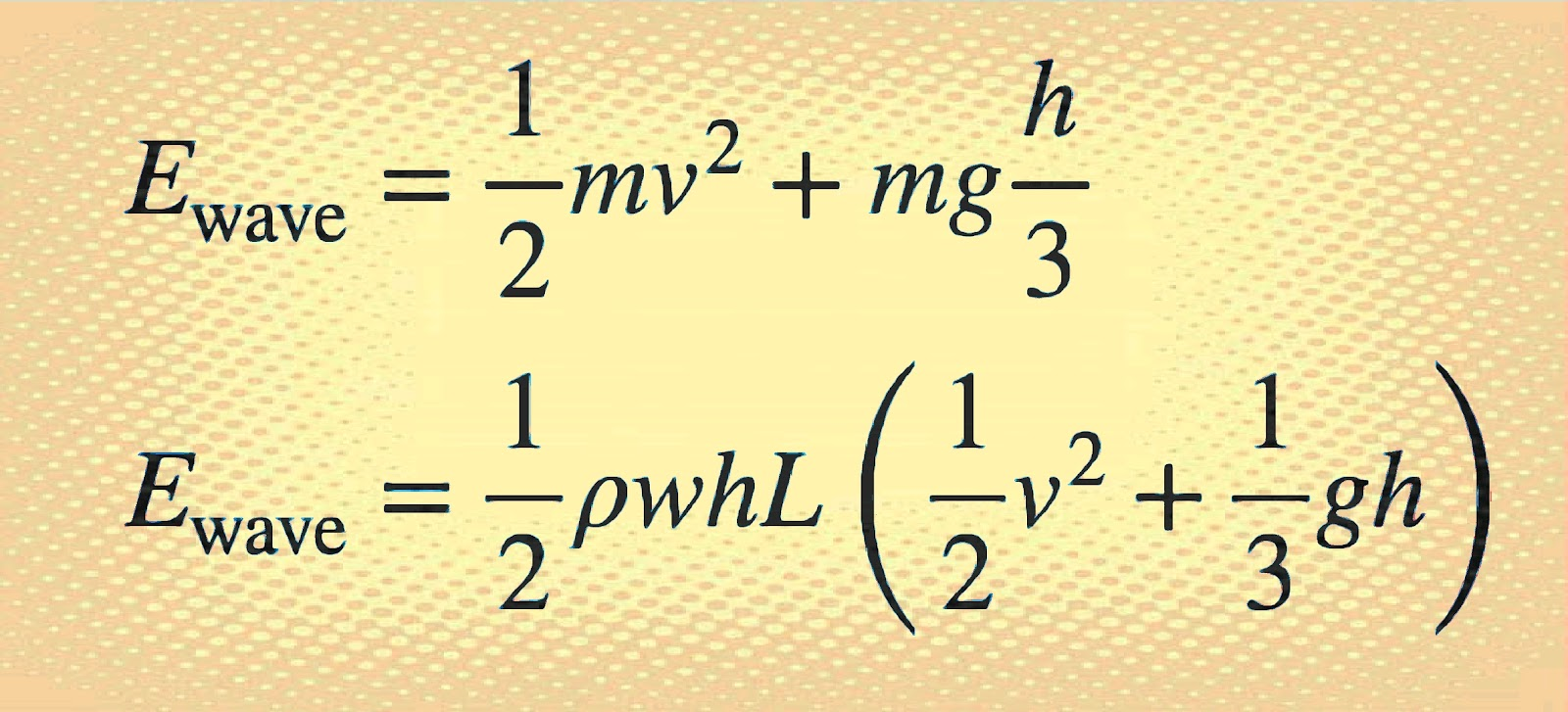That expression doesn’t look good, but at least now the energy calculation is in terms of things that we actually know or can estimate.

All we have to do is convert our estimates from imperial units to metric and we’re good to go.

Using a wave traveling at 20 miles per hour, with these estimates I get a wave energy of 16 million joules.

Is This A Lot Of Energy?

Here are some quick numbers to compare.

Suppose you take a textbook off the floor and put it on a table.

This takes approximately 10 joules.

Your smartphone battery stores about 10.000 joules. A full tank of gasoline, or 12 gallons, equals about 1.5 billion joules.

Ok, now that we know the energy it takes to make a wave, we have a few options on how to create this thing. Suppose you use an electric motor to pull the hydrofoil.

If the motor is 85 percent efficient, you’d actually need to put 19 million joules into it to get 16 million joules out of the wave.

The average price of electricity in the US is 23 cents per kilowatt-hour.  Power is a measure of how fast you use energy, and we can calculate it as P = E / ΔT , where T is time.  If energy is in joules and time is in seconds, then power would be in watts.

So 1 kilowatt-hour is the power you’d get running 1.000 watts for 1 hour (3.600 seconds), or 3.6 million joules.

That’s how much power you get for just 23 cents.

If you want 19 million joules, it would cost you \$1.23.

What about a gas powered hydrofoil?

In the US, you typically buy gasoline by the gallon; in other parts of the world, it is sold by the liter.

Gasoline stores about 34 million joules per liter (or 128 million joules per gallon).

However, a gasoline engine is much less efficient than an electric motor.

At best, it would be 40 percent efficient.

That means we would need to use 40.9 million joules, or 1.2 liters (0.32 gallons) of gasoline.

Assuming you pay \$3 per gallon (which is slightly lower than the US average as of July 2023), that would cost close to \$1, or about the same price as an electrically generated wave.

Now, let’s say you’re really off the grid and want to make waves with human power.

Obviously you can’t pull hydrofoil by yourself.

But maybe you could pedal a bike to lift a large mass using pulleys, and once the mass had enough energy, you’d drop it and pull the hydrofoil.

Let’s say this entire system is 50 percent efficient, so you would need to produce enough power to store 32 million joules.

Suppose you can generate 100 watts of power.

How long would it take you to store that much energy for your wave?.

Let’s calculate this:

The Energy Stored Equation

That’s about 89 hours to store that amount of energy. Even if you work 10-hour shifts, it will take you more than nine days to get enough power for a single wave.

Technically, this wave is priceless, but it will still cost you.

At least if you’re riding your waves this way, you’ll have plenty of time to think about your bad decisions as you prepare for the next surf session.

I would probably choose electric waves instead.

SYNTHESIS:

There is a sustainable solution for every need, although the adventure of riding a natural wave will never be comparable to contact with nature.

Technology well used shows that it can solve everyday problems.

CONCLUSION:

With this mathematical demonstration, an attempt was made to demonstrate how renewable energies can be used even to satisfy such natural needs as leisure or sports, “natural but artificial sports, with renewable energy”.

This was a commissioned essay for the University of Hawaii and CleanTech.

AUTHOR:

DIEGO BALVERDE

ECONOMIST

EUROPEAN CENTRAL BANK